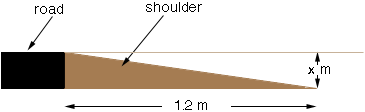SEARCH HOMEMath Central Quandaries & QueriesQuestion from Robert: I am building a road with a 1.2 meter shoulder. The plan calls for a 6% shoulder grade. When I use my metric calculator I come up with a different answer when I multiply 3/4 of an inch by 1.2 meters and when I multiply 1.2 meters by .06%?Hi Robert,

Your shoulder is 1.2 m wide and the grade is to be 6% so if x is as in the diagram then x/1.2 = 0.06.Thus x = 1.20.06 = 0.072 m or 0.072100 = 7.2 cm. Since there are 2.54 cm in an inch this is

7.2/2.54 = 2.83 inches.

I hope this helps,
PennyMath Central is supported by the University of Regina and The Pacific Institute for the Mathematical Sciences.